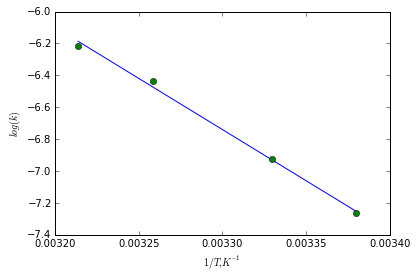# Chapter 18: Elementary Chemical Kinetics¶

## Example Problem 18.2, Page Number 451¶

In :
from math import log

#Variable Declaration
Ca0 = [2.3e-4,4.6e-4,9.2e-4]       #Initial Concentration of A, M
Cb0 = [3.1e-5,6.2e-5,6.2e-5]       #Initial Concentration of B, M
Ri  = [5.25e-4,4.2e-3,1.68e-2]     #Initial rate of reaction, M

#Calculations
alp = log(Ri/Ri)/log(Ca0/Ca0)
beta = (log(Ri/Ri) - 2*log((Ca0/Ca0)))/(log(Cb0/Cb0))
k = Ri/(Ca0**2*Cb0**beta)

#REsults
print 'Order of reaction with respect to reactant A: %3.2f'%alp
print 'Order of reaction with respect to reactant A: %3.2f'%beta
print 'Rate constant of the reaction: %4.3e 1./(M.s)'%k

Order of reaction with respect to reactant A: 2.00
Order of reaction with respect to reactant A: 1.00
Rate constant of the reaction: 3.201e+08 1./(M.s)


## Example Problem 18.3, Page Number 457¶

In :
from math import log

#Variable Declaration
t1by2 = 2.05e4       #Half life for first order decomposition of N2O5, s
x = 60.              #percentage decay of N2O5

#Calculations
k = log(2)/t1by2
t = -log(x/100)/k

#REsults
print 'Rate constant of the reaction: %4.3e 1/s'%k
print 'Timerequire for 60 percent decay of N2O5: %4.3e s'%t

Rate constant of the reaction: 3.381e-05 1/s
Timerequire for 60 percent decay of N2O5: 1.511e+04 s


## Example Problem 18.4, Page Number 457 Incomplete¶

In [ ]:
from math import log

#Variable Declaration
t1by2 = 5760       #Half life for C14, years

#Calculations
k = log(2)/t1by2
t = -log(x/100)/k

#REsults
print 'Rate constant of the reaction: %4.3e 1/s'%k
print 'Timerequire for 60 percent decay of N2O5: %4.3e s'%t


## Example Problem 18.5, Page Number 463¶

In :
from math import log

#Variable Declaration
kAbykI = 2.0       #Ratio of rate constants
kA = 0.1           #First order rate constant for rxn 1, 1/s
kI = 0.05          #First order rate constant for rxn 2, 1/s
#Calculations
tmax = 1/(kA-kI)*log(kA/kI)

#Results
print 'Time required for maximum concentration of A: %4.2f s'%tmax

Time required for maximum concentration of A: 13.86 s


## Example Problem 18.7, Page Number 467¶

In :
from math import log

#Variable Declaration
T = 22.0           #Temperature of the reaction,°C
k1 = 7.0e-4        #Rate constants for rxn 1, 1/s
k2 = 4.1e-3        #Rate constant for rxn 2, 1/s
k3 = 5.7e-3        #Rate constant for rxn 3, 1/s
#Calculations
phiP1 = k1/(k1+k2+k3)

#Results
print 'Percentage of Benzyl Penicillin that under acid catalyzed reaction by path 1: %4.2f '%(phiP1*100)

Percentage of Benzyl Penicillin that under acid catalyzed reaction by path 1: 6.67


## Example Problem 18.8, Page Number 468¶

In :
from numpy import arange,array,ones,linalg,log, exp
from matplotlib.pylab import plot, show
%matplotlib inline

#Variable Declaration
T = array([22.7,27.2,33.7,38.0])
k1 = array([7.e-4,9.8e-4,1.6e-3,2.e-3])
R = 8.314

#Calculations
T = T +273.15
x = 1./T
y = log(k1)
A = array([ x, ones(size(x))])
# linearly generated sequence
[slope, intercept] = linalg.lstsq(A.T,y) # obtaining the parameters

# Use w and w for your calculations and give good structure to this ipython notebook
# plotting the line
line = slope*x+intercept # regression line
#Results
plot(x,line,'-',x,y,'o')
xlabel('$1/T, K^{-1}$')
ylabel('$log(k)$')
show()
Ea = -slope*R
A = exp(intercept)
print 'Slope and intercept are, %6.1f and %4.2f'%(slope, intercept)
print 'Pre-exponential factor and Activation energy are %4.2f kJ/mol and %4.2e 1/s'%(Ea/1e3, A)Slope and intercept are, -6419.8 and 14.45
Pre-exponential factor and Activation energy are 53.37 kJ/mol and 1.88e+06 1/s


## Example Problem 18.9, Page Number 473¶

In :
from math import exp

#Variable Declaration
Ea = 42.e3      #Activation energy for reaction, J/mol
A  = 1.e12      #Pre-exponential factor for reaction, 1/s
T = 298.0       #Temeprature, K
Kc = 1.0e4      #Equilibrium constant for reaction
R = 8.314       #Ideal gas constant, J/(mol.K)
#Calculations
kB = A*exp(-Ea/(R*T))
kA = kB*Kc
kApp = kA + kB

#Results
print 'Forward Rate constant is %4.2e 1/s'%kA
print 'Backward Rate constant is %4.2e 1/s'%kB
print 'Apperent Rate constant is %4.2e 1/s'%kApp

Forward Rate constant is 4.34e+08 1/s
Backward Rate constant is 4.34e+04 1/s
Apperent Rate constant is 4.34e+08 1/s


## Example Problem 18.10, Page Number 480¶

In :
from math import pi
#Variable Declaration
Dh = 7.6e-7     #Diffusion coefficient of Hemoglobin, cm2/s
Do2 = 2.2e-5    #Diffusion coefficient of oxygen, cm2/s
rh = 35.        #Radius of Hemoglobin, °A
ro2 = 2.0       #Radius of Oxygen, °A
k = 4e7         #Rate constant for binding of O2 to Hemoglobin, 1/(M.s)
#Calculations
DA = Dh + Do2
kd = 4*pi*NA*(rh+ro2)*1e-8*DA

#Results
print 'Estimated rate %4.1e 1/(M.s) is far grater than experimental value of %4.1e 1/(M.s), \nhence the reaction is not diffusion controlled'%(kd,k)

Estimated rate 6.4e+13 1/(M.s) is far grater than experimental value of 4.0e+07 1/(M.s),
hence the reaction is not diffusion controlled


## Example Problem 18.11, Page Number 484¶

In :
from math import log, e
#Variable Declaration
Ea = 104e3      #Activation energy for reaction, J/mol
A  = 1.e13      #Pre-exponential factor for reaction, 1/s
T = 300.0       #Temeprature, K
R = 8.314       #Ideal gas constant, J/(mol.K)
h = 6.626e-34   #Plnak constant, Js
c = 1.0         #Std. State concentration, M
k = 1.38e-23    #,J/K

#Calculations
dH = Ea - 2*R*T
dS = R*log(A*h*c/(k*T*e**2))

#Results
print 'Forward Rate constant is %4.2e 1/s'%dH
print 'Backward Rate constant is %4.2f 1/s'%dS

Forward Rate constant is 9.90e+04 1/s
Backward Rate constant is -12.72 1/s